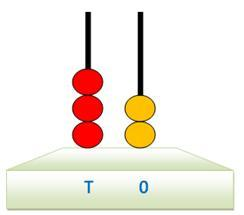# Two-digit 3456

Write all the two-digit numbers that can be composed of the digit 7,8,9 without repeating the digits. Which ones are divisible
b) two,
c) three
d) six?

Result

a = (Correct answer is: 78,79,87,89,97,98)b = (Correct answer is: 78,98)c = (Correct answer is: 78,87)d = (Correct answer is: 78)### Step-by-step explanation:Did you find an error or inaccuracy? Feel free to write us. Thank you!

Tips for related online calculators